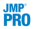Publication date: 07/30/2020

## Validation Method

Neural networks are very flexible models and have a tendency to overfit data. When that happens, the model predicts the fitted data very well, but predicts future observations poorly. To mitigate overfitting, the Neural platform does the following:

applies a penalty on the model parameters

uses an independent data set to assess the predictive power of the model

Validation is the process of using part of a data set to estimate model parameters, and using the other part to assess the predictive ability of the model.

The training set is the part that estimates model parameters.

The validation set is the part that estimates the optimal value of the penalty, and assesses or validates the predictive ability of the model.

The test set is a final, independent assessment of the model’s predictive ability. The test set is available only when using a validation column.

The training, validation, and test sets are created by subsetting the original data into parts. Select one of the following methods to subset a data set.

Excluded Rows Holdback

Uses row states to subset the data. Rows that are unexcluded are used as the training set, and excluded rows are used as the validation set.

For more information about using row states and how to exclude rows, see Hide and Exclude Rows in Using JMP.

Holdback

Randomly divides the original data into the training and validation sets. You can specify the proportion of the original data to use as the validation set (holdback).

KFold

Divides the original data into K subsets. In turn, each of the K sets is used to validate the model fit on the rest of the data, fitting a total of K models. The model giving the best validation statistic is chosen as the final model.

This method is best for small data sets, because it makes efficient use of limited amounts of data.Validation Column

Uses the column’s values to divide the data into parts. The column is assigned using the Validation role on the Neural launch window (Figure 3.3).

The column’s values determine how the data is split, and what method is used for validation:

If the column has three unique values, then:

the smallest value is used for the Training set.

the middle value is used for the Validation set.

the largest value is used for the Test set.

If the column has two unique values, then only Training and Validation sets are used.

If the column has more than three unique values, then K-Fold validation is performed.